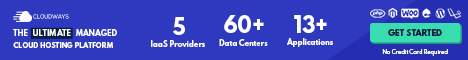# The Aggregate-Supply Curve

The aggregate-supply curve tells us the total quantity of goods and services that firms produce and sell at any given price level. The upward-sloping aggregate supply curve shows the relationship between the price level and the level of real GDP.

The curve slopes up because when the price level for outputs increases, while the price level of inputs remains fixed, the opportunity for additional profits encourages more production.

The aggregate supply curve is near-horizontal on the left and near-vertical on the right. A vertical line near the right-hand side of the aggregate-supply curve shows the level of potential GDP, which is the maximum level of output the economy can produce with its existing levels of workers, physical capital, technology, and economic institutions.Figure I, The Aggregate-Supply Curve | Source: EconomicsKey.com Opens in new window

Unlike the aggregate-demand curve Opens in new window, which always slopes downward, the aggregate-supply curve shows a relationship that depends crucially on the time horizon examined.

In the long run, the aggregate-supply curve is vertical, whereas in the short run, the aggregate-supply curve slopes upward.

To understand short-run economic fluctuations Opens in new window, and how the short-run behavior of the economy Opens in new window deviates from its long-run behavior, we need to examine both the long-run aggregate-supply curve and the short-run aggregate supply curve.

## Why the Aggregate-Supply Curve Is Vertical in the Long Run

What determines the quantity of goods and services supplied in the long run?

We implicitly answered this question earlier in the series when we analyzed the process of economic growth Opens in new window.

In the long run Opens in new window, an economy’s production of goods and services (its real GDP) depends on its supplies of labor, capital, and natural resources and on the available technology used to turn these factors of production into goods and services.Because the price level does not affect the long-run determinants of real GDP, the long-run aggregate-supply curve is vertical, as shown in Figure I.

In other words, in the long run, the economy’s labor, capital, natural resources, and technology determine the total quantity of goods and services supplied, and this quantity supplied is the same regardless of what the price level happens to be.

The vertical long-run aggregate-supply curve is a graphical representation of the classical dichotomy and monetary neutrality. Classical macroeconomic theory is based on the assumption that real variables do not depend on nominal variables.

The long-run aggregate-supply curve is consistent with this idea because it implies that the quantity of output (a real variable) does not depend on the level of prices (a nominal variable).

Most economists believe this principle works well when studying the economy over a period of many years but not when studying year-to-year changes. Thus, the aggregate-supply curve is vertical only in the long run.